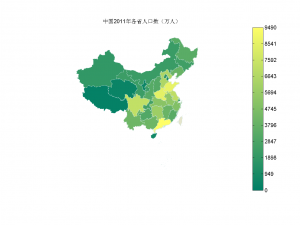``````%-- 分省中国地图在MATLAB中的画法 --%
% Author: gaospecial@gmail.com
% Date: 2012-6-5 14:04:01
% 地图数据来源：国家基础地理信息系统网站
% 人口数据来源：国家统计年鉴2011版
``````

## 载入地图数据

``````sheng=shaperead('maps/bou2_4p.shp', 'UseGeoCoords', true); % 省
% 检查数据发现中午名称为乱码，可能是由于字符编码引起的，需要纠正文件导入时出现的乱码
% 中文名称记录在相应的dbf文件中，可以使用Excel打开，并且不会出现乱码，截取其中的中文保存为文本文件
% 然后在导入工作区，并将其保存为*.mat格式文件，随用随取。
% 依次替换sheng数据中的乱码【算是变通之法，有谁知道更好的方法一定告诉我哦】
for i=1:length(sheng)
sheng(i).NAME=sheng_chinese_name{i}; % 纠正中文显示错误
end
unique(sheng_chinese_name) % 含有34个省（直辖市）的数据
length(sheng) % 共分为925个区块
``````
``````ans =

'上海市'
'云南省'
'内蒙古自治区'
'北京市'
'台湾省'
'吉林省'
'四川省'
'天津市'
'宁夏回族自治区'
'安徽省'
'山东省'
'山西省'
'广东省'
'广西壮族自治区'
'新疆维吾尔自治区'
'江苏省'
'江西省'
'河北省'
'河南省'
'浙江省'
'海南省'
'湖北省'
'湖南省'
'甘肃省'
'福建省'
'空'
'西藏自治区'
'贵州省'
'辽宁省'
'重庆市'
'陕西省'
'青海省'
'香港特别行政区'
'黑龙江省'

ans =

925
``````

## 导入2011年各省人口数据

``````d=importdata('renkou.txt');
data=d.data; % 人口数目
textdata=d.textdata; % 相对应的省的名称
``````

## 定义地图参数

``````k=128;
mycolormap=summer(k);
% 生成不同区域按大小的颜色，按照人口数目多少分别指定不同的颜色
% 人口越多，颜色越突出
geoname={sheng.NAME}';
max_data = max(data);
n=length(data);
mysymbolspec=cell(1,n);% 预定义变量可以加快处理速度
for i=1:n
count=data(i);
mycoloridx=floor( k * count / max_data );
mycoloridx(mycoloridx<1)=1;
myprovince=textdata{i};
geoidx=strmatch(myprovince, geoname);
if numel(geoidx) > 0
province_name=geoname( geoidx(1) );
mysymbolspec{i} = {'NAME', char(province_name), 'FaceColor', mycolormap( mycoloridx, :) };
end
end
``````

## 显示地图

``````figure
ax=worldmap('china'); % 使用worldmap的坐标轴作图
setm(ax,'grid','off') % 关闭grid
setm(ax,'frame','off') % 关闭边框
setm(ax,'parallellabel','off') % 关闭坐标轴标记
setm(ax,'meridianlabel','off') % 关闭坐标轴标记

% 最关键的两个语句
symbols=makesymbolspec('Polygon',{'default','FaceColor',[0.9 0.9 0.8],...
'LineStyle,'--','LineWidth',0.2,...
'EdgeColor',[0.8 0.9 0.9]},...
mysymbolspec{:}...
);
geoshow(sheng,'SymbolSpec',symbols); % 此处用mapshow投影会不正确
``````

## 图的标注

``````% 在图像右侧显示bar
colormap(summer(k))
hcb=colorbar('EastOutside');
step=round(max_data/11);
set(hcb,'YTick',(0:.1:1))
set(hcb,'YTickLabel',num2cell(0:step:max_data))

% 给图像加标题
title('中国2011年各省人口数（万人）')
``````

china_map

### 作者简介

Chun-Hui Gao is a Research Associate at Huazhong Agricultural University.

### 引用本文

``gaoch (2012). MatLab中国地图进阶：以不同的颜色表示各省数据的差异. BIO-SPRING. https://bio-spring.top/post/2012/06/05/2012-06-05-matlab-chinamap-coloring/``

BibTeX citation

``````@misc{
title = "MatLab中国地图进阶：以不同的颜色表示各省数据的差异",
author = "gaoch",
year = "2012",
journal = "BIO-SPRING",
note = "https://bio-spring.top/post/2012/06/05/2012-06-05-matlab-chinamap-coloring/"
}``````